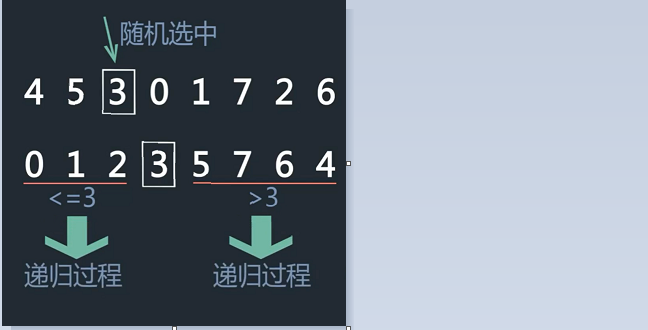# 排序算法总结

### 排序算法

#### 一、算法概述

1.冒泡排序 O(N2)，空间O(1)

2.选择排序O(N2)，空间O(1)

3.堆排序O(NlogN)，空间O(1)

public class HeapSort {
public static void main(String[] args) {
int[] array = new int[] { 2, 1, 4, 3, 6, 5, 8, 7 };
// 接下来就是排序的主体逻辑
sort(array);
System.out.println(Arrays.toString(array));
}
public static void sort(int[] array) {

for (int i = array.length / 2 - 1; i >= 0; i--) {
}

for (int j = array.length - 1; j > 0; j--) {

}
}

public static void adjustHeap(int[] array, int i, int length) {

int temp = array[i];

for (int k = 2 * i + 1; k < length; k = 2 * k + 1) {

if (k + 1 < length && array[k] < array[k + 1]) {
k++;
}

if (array[k] > temp) {
swap(array, i, k);

i = k;

} else {
break;
}
}
}

public static void swap(int[] arr, int a, int b) {
int temp = arr[a];
arr[a] = arr[b];
arr[b] = temp;
}
}


4.插入排序O(N2)，空间O(1)

5.希尔排序O(?)，空间O(1)

k每轮减1，一直到1，这样做其实利用插入排序的优势。

6.归并排序O(NlogN)，空间O(N)

7.快速排序O(NlogN)，空间O(logN)主要难写的就是划分区域的部分


（各扫到一个不满足条件的数后，交换，进行下一轮）

   temp = a[left]; //temp中存的就是基准数
i = left;
j = right;
while(i != j) { //顺序很重要，要先从右边开始找
while(a[j] > temp && i < j)
j--;
while(a[i] <= temp && i < j)//再找左边的
i++;
if(i < j)//交换两个数在数组中的位置
{
t = a[i];
a[i] = a[j];
a[j] = t;
}
}
//最终将基准数归位
a[left] = a[i];
a[i] = temp;



8.桶排序O(N)，空间O(M)

• 计数排序

数字区间范围小的，比如年龄、身高

• 基数排序

以数字为0-9作为桶，从低位到高位排

posted @ 2019-07-14 12:27  hzhuan  阅读(...)  评论(...编辑  收藏